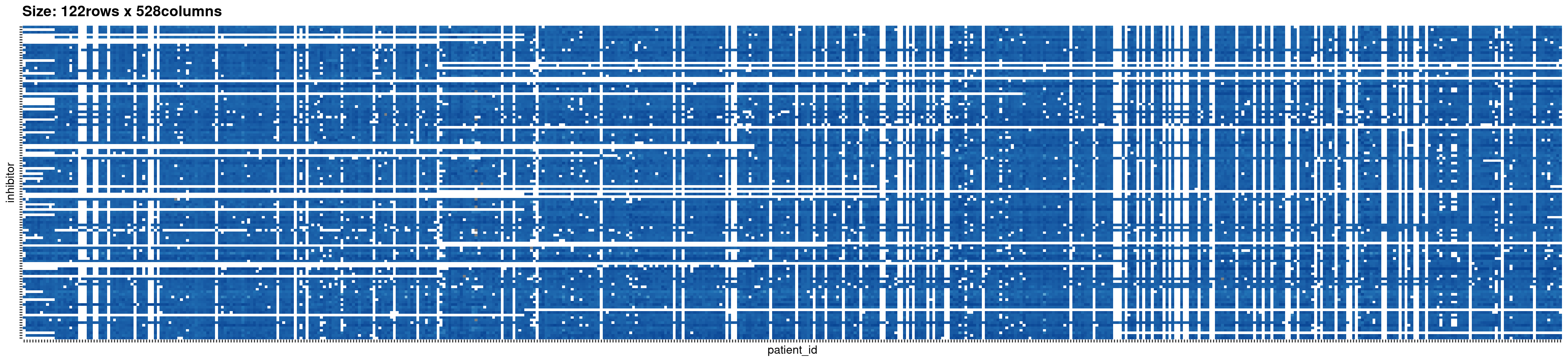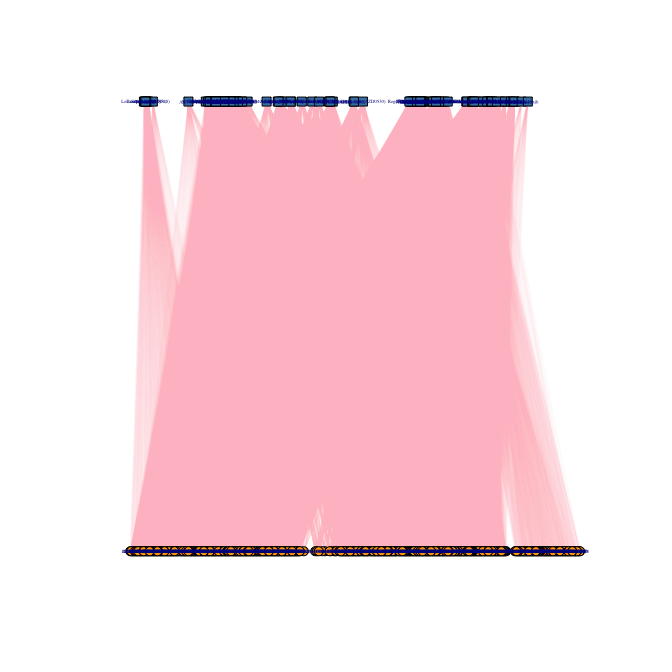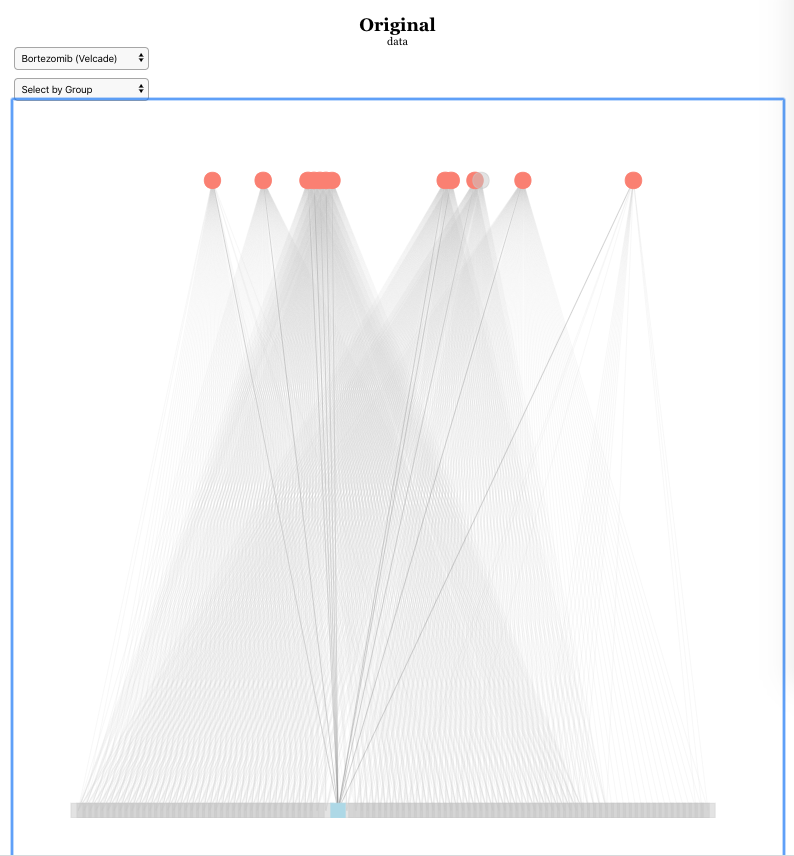# NIMAA-vignette

## Introduction

Many numerical analyses are invalid when working with nominal data because the mode is the only way to measure central tendency for nominal data, and frequency testing, like Chi-square tests, is the most common statistical analysis that makes sense. `NIMAA` package (Jafari and Chen 2022) proposes a comprehensive set of pipeline to perform nominal data mining, which can effectively find label relationships of each nominal variable according to pairwise association with other nominal data. You can also check for updates, here NIMAA.

It uses bipartite graphs to show how two different types of data are linked together, and it puts them in the incidence matrix to continue with network analysis. Finding large submatrices with non-missing values and edge prediction are other applications of NIMAA to explore local and global similarities within the labels of nominal variables.

Then, using a variety of different network projection methods, two unipartite graphs are constructed on the given submatrix. NIMAA provides several options for clustering projected networks and selecting the best one based on internal measures and external prior knowledge (ground truth). When weighted bipartite networks are considered, the best clustering results are used as the benchmark for edge prediction analysis. This benchmark is used to figure out which imputation method is the best one to predict weight of edges in bipartite network. It looks at how similar the clustering results are before and after the imputations. By using edge prediction analysis, we tried to get more information from the whole dataset even though there were some missing values.

``library(NIMAA)``

## 1 Exploring the data

In this section, we demonstrate how to do a NIMAA analysis on a weighted bipartite network using the `beatAML` dataset. `beatAML` is one of four datasets that can be found in the `NIMAA` package (Jafari and Chen 2022). This dataset has three columns: the first two contain nominal variables, while the third contains numerical variables.

The first ten rows of beatAML dataset
inhibitor patient_id median
Alisertib (MLN8237) 11-00261 81.00097
Barasertib (AZD1152-HQPA) 11-00261 60.69244
Canertinib (CI-1033) 11-00261 87.03067
Crenolanib 11-00261 68.13586
CYT387 11-00261 69.66083
Dasatinib 11-00261 66.13318
Doramapimod (BIRB 796) 11-00261 101.52120
Dovitinib (CHIR-258) 11-00261 33.48040
Erlotinib 11-00261 56.11189

Read the data from the package:

``````# read the data
beatAML_data <- NIMAA::beatAML``````

### 1.1 Plotting the original data

The `plotIncMatrix()` function prints some information about the incidence matrix derived from input data, such as its dimensions and the proportion of missing values, as well as the image of the matrix. It also returns the incidence matrix object.

NB: To keep the size of vignette small enough for CRAN rules, we won’t output the interactive figure here.

``````beatAML_incidence_matrix <- plotIncMatrix(
x = beatAML_data, # original data with 3 columns
index_nominal = c(2,1), # the first two columns are nominal data
index_numeric = 3,  # the third column is numeric data
print_skim = FALSE, # if you want to check the skim output, set this as TRUE
plot_weight = TRUE, # when plotting the weighted incidence matrix
verbose = FALSE # NOT save the figures to local folder
)``````

Na/missing values Proportion: 0.2603The beatAML dataset as an incidence matrix

### 1.2 Plotting the bipartite network of the original data

Given that we have the incidence matrix, we can easily reconstruct the corresponding bipartite network. In the `NIMAA` package, we have two options for visualizing the bipartite network: static or interactive plots.

#### 1.2.1 Static plot

The `plotBipartite()` function customizes the corresponding bipartite network visualization based on the `igraph` package (Csardi and Nepusz 2006) and returns the igraph object.

``bipartGraph <- plotBipartite(inc_mat = beatAML_incidence_matrix, vertex.label.display = T)````````
# show the igraph object
bipartGraph
#> IGRAPH bd183bf UNWB 650 47636 --
#> + attr: name (v/c), type (v/l), shape (v/c), color (v/c), weight (e/n)
#> + edges from bd183bf (vertex names):
#>   Alisertib (MLN8237)      --11-00261 Barasertib (AZD1152-HQPA)--11-00261
#>   Bortezomib (Velcade)     --11-00261 Canertinib (CI-1033)     --11-00261
#>   Crenolanib               --11-00261 CYT387                   --11-00261
#>   Dasatinib                --11-00261 Doramapimod (BIRB 796)   --11-00261
#>   Dovitinib (CHIR-258)     --11-00261 Erlotinib                --11-00261
#>  Flavopiridol             --11-00261 GDC-0941                 --11-00261
#>  Gefitinib                --11-00261 Go6976                   --11-00261
#>  GW-2580                  --11-00261 Idelalisib               --11-00261
#> + ... omitted several edges``````

#### 1.2.2 Interactive plot

The `plotBipartiteInteractive()` function generates a customized interactive bipartite network visualization based on the `visNetwork` package (Almende B.V. and Contributors, Thieurmel, and Robert 2021).

NB: To keep the size of vignette small enough, we do not output the interactive figure here. Instead, we show a screenshot of part of the `beatAML` dataset.

``plotBipartiteInteractive(inc_mat = beatAML_incidence_matrix)``beatAML dataset as incidence matrix

### 1.3 Analysis of network properties

`NIMAA` package contains a function called `analyseNetwork` to provide more details about the network topology and common centrality measures for vertices and edges.

``````analysis_reuslt <- analyseNetwork(bipartGraph)

# showing the general measures for network topology
analysis_reuslt\$general_stats
#> \$vertices_amount
#>  650
#>
#> \$edges_amount
#>  47636
#>
#> \$edge_density
#>  0.2258433
#>
#> \$components_number
#>  1
#>
#> \$eigen_centrality_value
#>  15721.82
#>
#> \$hub_score_value
#>  247175684``````

## 2 Extracting large submatrices without missing values

In the case of a weighted bipartite network, the dataset with the fewest missing values should be used for the next steps. This is to avoid the sensitivity problems of clustering-based methods. The `extractSubMatrix()` function extracts the submatrices that have non-missing values or have a certain percentage of missing values inside (not for elements-max matrix), depending on the argument’s input. The result will also be shown as a `plotly` plot (Sievert 2020), so you can see the screenshots of `beatAML` dataset below.

The extraction process is performed and visualized in two ways, which can be chosen depending on the user’s preference: using the original input matrix (row-wise) and using the transposed matrix (column-wise). `NIMAA` extracts the largest submatrices with non-missing values or with a specified proportion of missing values (using the `bar` argument) in four ways predefined in the `shape` argument:

• Square, to extract square matrix with the equal number of rows and columns,
• Rectangular_row, to extract matrix with the maximum number of rows,
• Rectangular_col, to extract matrix with the maximum number of columns,
• Rectangular_element_max, to extract matrix with the maximum number of elements.

Here we extract two shapes of submatrix from the `beatAML_incidence_matrix` including square and rectangular, with the maximum number of elements:

``````sub_matrices <- extractSubMatrix(
x = beatAML_incidence_matrix,
shape = c("Square", "Rectangular_element_max"), # the selected shapes of submatrices
row.vars = "patient_id",
col.vars = "inhibitor",
plot_weight = TRUE,
print_skim = FALSE
)
#> binmatnest2.temperature
#>                20.12122
#> Size of Square:   96 rows x  96 columns
#> Size of Rectangular_element_max:      87 rows x  140 columns``````
NB: To keep the size of vignette small enough, we show a screenshot.